# Good Definitions as Biconditionals; Polygons

### Union and Intersections

In Geometry, we will primarily be forming the unions and intersections of points, [line] segments, lines, and planes. Thus the sets will often be of infinite extent and thus their elements will often be referred to collectively and symbolically. An example would be the [line] segment (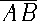) or line (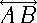) between or beyond points A and B. Please review especially the terms union, intersection, element, subset, null or empty set and their associated symbols: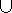,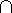,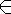,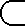,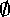or {} covered earlier in Numbers Lesson 1.

### Statements and Conjectures

In Geometry we must define things and often generate proofs. Some geometries state each definition in the form of a biconditional. What we prove are often logical statements in the form if-then and we must follow the rules of logic to form these proofs. Please review especially the symbols for conditional, converse, inverse, and contrapositive: p=>q, q=>p, ~p=>~q, ~q=>~p and terms statement, conjecture, biconditional, antecedent (hypothesis), consequent (conclusion) covered earlier in Numbers Lesson 5.

 An instance of a conditional p=>q is a case in which both p and q are true.

 A counterexample of a conditional p=>q is when p is true, but q is false.

Since to be true, a conditional must be true for every situation where p is true, sometimes, instead of writing a long proof, we will try to find a counterexample and prove it false.

An "if and only if" (often abbreviated iff) statement is called a biconditional and combines the statements p=>q and q=>p into p<=>q. To prove a biconditional, one proves the two corresponding conditionals.

 An example of the definition of union written as a biconditional is as follows: C=AB if and only if each element of C is in A, in B, or in both A and B.

Remember, a conditional and its contrapositive are either both true or both false. A common mistake is to assume this for the converse. This logic error is known as the fallacy of the converse.

Also, it is important to omit the if and the then when stating the antecedent (hypothesis) and the consequent (conclusion) of a given conditional. Often, the then is omitted entirely and/or the order of the antecedent and consequent are reversed: If it snows tomorrow, we will not have school. OR We will not have school tomorrow, if it snows. Watch out!

### Good Definitions

The textbook motivates the need for good definitions by citing a lawsuit involving dual textured cookies. In this suit Proctor & Gamble won \$125,000,000 from Nabisco, Keebler, and Frito-Lay. A careful definition of cookie was very important to the companies involved. A search of the Food and Drug Administration web site did not turn up a definition of cookie! A problem word for the United Nations and at least one Congressional Committee was the word terrorist. In 1964 the U.S. Supreme Court struggled with the definition of obscenity (hard-core pornography), resulting in Justice Stewart proclaiming after a movie day "I shall not today attempt further to define the kinds of material I understand to be embraced ... [b]ut I know it when I see it."

I should also remind the reader that certain words are purposely left undefined or perhaps defined by usage. Point, line, and plane were listed in chapter 1 along with the postulates which constraint them. Other terms, such as segment, or line segment were also defined there. Our text does not use the qualifier line before segment, but then it also does not define segment as the region between a chord and a circle. Please be flexible both in the name and what it represents. A good definition must:

1. include only words commonly understood, defined earlier, or purposely undefined;
2. accurately describe the idea being defined; and

Here are some examples. You should try rewriting these in biconditional form.

 The midpoint of a segment, is the point M onwith AM=MB.

 The bisector of a segment is a line, ray, or segment that intersects the segment at its midpoint.

Note that a bisector need not be perpendicular.

 A circle is the set of points in a plane equidistant from one point, its center. That distance is its radius.

A chord is a [line] segment with endpoints on the circle. The diameter of a circle is twice the radius. The diameter is also a chord containing the center. It thus may refer to either a distance or a set of points with that distance. Radius likewise is so used. Circles which have the same center (but perhaps different radii) are concentric. The interior of a circle is the set of points whose distance from the center is less than the radius. Notice that the circle is only the set of points on the circumference (outside the interior!) and does not include the points "inside" the circle. The region inside together with the circumference constitute a disk or disc. A circle also has an exterior. Study these definitions carefully. (Figures can be see in lesson 8.) Be sure each fulfills the qualities of a good definition. A good habit is to put all these definitions in your notes. Another good vocabulary word here is locus, a set of points determined by given conditions. The plural is loci. This is pronounced with either an si, ki, or ke sound.

### Convex, Nonconvex (Concave), etc.

 A convex set is a set in which every segment connecting points of the set lies entirely in the set.

If a set is not convex it is termed nonconvex. A polygon is convex if and only if its corresponding polygonal region is convex. We may use the word concave to describe a nonconvex polygon (but not a nonconvex set of points). Another term is re-entrant polygon. An easy way to remember the difference between convex and concave polygons is to think of a polygon with a side caved or dented in. Note that the set of points comprising any polygon are nonconvex. The term concave is more common in physics and is used in reference to lenses. The term nonconvex is the more precise mathematical term. However, our textbook doesn't even note the irony of a convex polygon being a nonconvex set of points. Beware!

### Polygons

 A polygon is the union of segments in the same plane such that each segment intersects exactly two others, one at each of its endpoints.

Notice that this definition allows polygons to be either concave or convex. However, a circle is not a polygon. A polygon also separates the plane into two disjoint regions, its interior and its exterior.

 The union of a polygon and its interior is a polygonal region.

If asked to sketch a polygonal region, be sure to shade the interior!

 A three-sided polygon is called a triangle.

Trigon is an old word for triangle which still appears in the word trigonometry. Notice that a [Euclidean] triangle can never be concave. A triangle with three equal sides is an equilateral triangle. One with at least two sides equal is an isosceles triangle. A Triangle with no sides equal is referred to as scalene. A triangle with a 90° angle is a right triangle. A triangle with all angles less than 90° is an acute triangle, and one with an angle greater than 90° is an obtuse triangle.

The Pythagorean Theorem states that a right triangle will have the sum of two sides squared equal to the square of the third side. One can tell with only side measurements if the triangle is acute, right or obtuse. If the sum of the squares of the two smallest sides is greater than the square of the third, then the triangle is acute. If it is less than, then it is obtuse.

 The sum of the length of two sides of a triangle is always greater than the third side.

This is known as the Triangle Inequality and is accepted as a postulate in this book. More information on special triangles, polygons, and trigonometry can be found in Numbers Lesson 11.

 A four-sided polygon is called a quadrilateral.

A quadrilateral that has opposite sides parallel is a parallelogram. Although we have defined parallel, we have not yet defined opposite! There are several other classifications for quadrilaterals: rhombus, kite, square, rectangle, and trapezoid. They will be defined formally in Geometry Lesson 6 along with the word apothem.

The term quadrangle is also used to refer to four-sided polygons, particularly certain grassy areas on college campuses bounded by buildings. An example on our campus would be the area with the flagpole(s) bounded by the library, seminary, church, and administration building.

Terms such as figures, polygon, triangle, etc. can be placed in a family tree or hierarchy. Generally, each type of figure is a special case of all types above it to which it is connected. We say it inherits those properties. Please note that this textbook assumes such a vertical orientation. (Note that such relationship charts are another example of networks.)

 A five-sided polygon is called a pentagon.

Our government's military headquarters were built in the shape of a pentagon and is thusly known.

 A six-sided polygon is called a hexagon.

Honeybees put honey in hexagonal prisms.

 A eight-sided polygon is called a octagon.

A stop sign has eight sides and is thus an octagon. Famous examples in archetecture are: Jefferson's Monticello and the Octagon House where President Madison lived after the White House was burned during the War of 1812 and he signed the treaty ending the war there. It should also be clear that heptagons have seven sides. nonagons have nine sides. Apparently, nonagons are occasionally but more properly called enneagons. (This is because of the mixing of Latin prefixes with Greek suffixes.) decagons have ten sides. The Pokagons are a local band of Native Americans of the Potawatomi tribe. If you search hard enough you might find: undecagon or hendecagon (11 sides), dodecagon or duodecagon (12 sides), tetradecagon (14 sides), quindecagon (15 sides), heptadecagon (17 sides--this one is important since it is constructable), enneadecagon (19 sides), icosagon (20 sides), hectagon (100 sides), and zonagon (even number of sides, all sides equal, opposite sides parallel).

 A n-sided polygon is called a n-gon.

 The segment intersection points in a polygon are termed vertices (plural) or vertex (singular).

 The segments which make up a polygon are termed sides.

 Consecutive or adjacent vertices are endpoints of the same side.

 Consecutive or adjacent sides are sides which share an endpoint.

 A diagonal is a segment connecting nonadjacent vertices.

 A general formula for the number of diagonals in an n-gon is n•(n-3)/2.

The formula above can easily be derived or remembered by noting that an n-gon has n vertices. Each vertex can connect to any other vertex except itself and the two adjacent vertices, hence the (n-3) factor. Each diagonal also has two endpoints and we must avoid double counting them, hence the 2 in the denominator.

Regular polygons were also discussed earlier in Numbers lesson 11. An equiangular polygon may also be termed a isogon.

Note that you can triangulate an arbitrary convex n-gon by dividing it into n-2 triangles. Do this by drawing all the diagonals possible from one vertex. From this and the fact that angles in a triangle sum to 180°, it can be derived that the sum of the angles in any n-gon is (n-2)•180°. We can then further discover that the measure of any angle in a regular polygon is (n-2)•180°÷n. Another item to relate the n-2 to is the fact that the exterior angles of a polygon all sum to 360°=2•180° and together the interior and exterior angles of an n-gon must sum to n•180°. Although true for concave polygons, the proof would be much more difficult.

Sometimes the question is asked as to how many triangles can be formed by intersecting diagonals in a regular polygon. Results up to n=20 are located here.

BACK HOMEWORK ACTIVITY CONTINUE